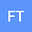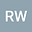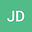•••This paper describes magnetospheric waves of very long wavelength in thin magnetic filaments. We consider an average magnetospheric configuration with zero ionospheric conductance and calculate waves using two different formulations: classic interchange theory and ideal MHD. Classic interchange theory, which is developed in detail in this paper, is basically analytic and is relatively straightforward to determine computationally, but it can’t offer very high accuracy.The two formalisms agree well for the plasma sheet and also for the inner magnetosphere. The eigenfrequencies range over about a factor of seven, but the formulations generally agree with a root-mean-square difference of the $log_{10}$ of the ratio of the interchange to MHD frequencies to be $\sim 0.054$. The pressure perturbations in the classic interchange theory are assumed constant along each field line, but the MHD computed pressure perturbations along the field line vary in a range $\sim 30 \%$ in the plasma sheet but are larger in the inner magnetosphere. The parallel and perpendicular displacements, which are very different in the plasma sheet and inner magnetosphere, show good qualitative agreement between the two approaches. In the plasma sheet, the perpendicular displacements are strongly concentrated in the equatorial plane, whereas the parallel displacements are spread through most of the plasma sheet away from the equatorial plane; and can be regarded as buoyancy waves. In the inner magnetosphere, the displacements are more sinusoidal and are more like conventional slow modes. The different forms of the waves are best characterized by the flux tube entropy $PV^\gamma$.